# NCERT Solutions For Class 12 Maths Chapter 12 Exercise 12.1

## Chapter 12 Ex.12.1 Question 1

Maximize $$Z = 3x + 4y$$

Subject to the constraints: $$x + y \le 4,\;x \ge 0,\;y \ge 0$$

### Solution

The feasible region determined by the constraints, $$x + y \le 4,\;x \ge 0$$ and $$y \ge 0$$ is given by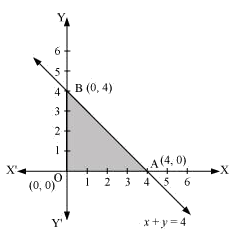Since, the corner points of the feasible region are $$O\left( {0,0} \right),A\left( {4,0} \right)$$ and $$B\left( {0,4} \right)$$. The value of $$Z$$ at these points are as follows:

 Corner point $$Z = 3x + 4y$$ $$O\left( {0,0} \right)$$ 0 $$A\left( {4,0} \right)$$ 12 $$B\left( {0,4} \right)$$ 16 $$\to$$Maximum

Thus, the maximum value of $$Z$$ is $$16$$ at the point $${\rm{B}}\left( {0,4} \right)$$.

## Chapter 12 Ex.12.1 Question 2

Minimize $$Z = - 3x + 4y$$

Subject to $$x + 2y \le 8,\;3x + 2y \le 12,\;x \ge 0,\;y \ge 0$$

### Solution

The feasible region determined by the system of constraints, $$x + 2y \le 8,\;3x + 2y \le 12,\;x \ge 0$$ and $$y \ge 0$$, is given by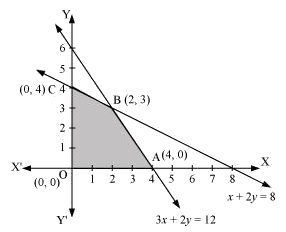Since, the corner points of the feasible region are $$O\left( {0,0} \right),A\left( {4,0} \right),B\left( {2,3} \right)$$ and $$C\left( {0,4} \right)$$. The value of $$Z$$ at these corner points are as follows:

 Corner point $$Z = - 3x + 4y$$ $${\rm{O}}\left( {0,0} \right)$$ 0 $${\rm{A}}\left( {4,0} \right)$$ $$- 12$$ $$\to$$Minimum $${\rm{B}}\left( {2,3} \right)$$ 6 $${\rm{C}}\left( {0,4} \right)$$ 16

Thus, the minimum value of $$Z$$ is $$- 12$$ at the point $${\rm{A}}\left( {4,0} \right)$$.

## Chapter 12 Ex.12.1 Question 3

Maximize $$Z = 5x + 3y$$

Subject to $$3x + 5y \le 15,\;5x + 2y \le 10,\;x \ge 0,\;y \ge 0$$

### Solution

The feasible region determined by the system of constraints $$3x + 5y \le 15,\;5x + 2y \le 10,\;x \ge 0$$ and $$y \ge 0$$, is given by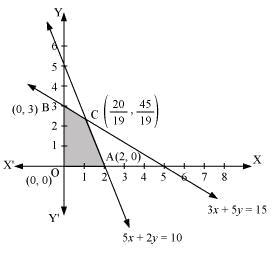Since, the corner points of the feasible region are $$O\left( {0,0} \right),A\left( {2,0} \right),B\left( {0,3} \right)$$ and $$C\left( {\frac{{20}}{{19}},\frac{{45}}{{19}}} \right)$$. The value of $$Z$$ at these corner points are as follows:

 Corner point $$Z = 5x + 3y$$ $${\rm{O}}\left( {0,0} \right)$$ 0 $${\rm{A}}\left( {2,0} \right)$$ 10 $${\rm{B}}\left( {0,3} \right)$$ 9 $${\rm{C}}\left( {\frac{{20}}{{19}},\frac{{45}}{{19}}} \right)$$ $$\frac{{235}}{{19}}$$ $$\to$$Maximum

Thus, the maximum value of $$Z$$ is $$\left( {\frac{{235}}{{19}}} \right)$$ at the point $${\rm{C}}\left( {\frac{{20}}{{19}},\frac{{45}}{{19}}} \right)$$.

## Chapter 12 Ex.12.1 Question 4

Minimize $$Z = 3x + 5y$$

Subject to $$x + 3y \ge 3,\;x + y \ge 2,\;x,\;y \ge 0$$

### Solution

The feasible region determined by the system of constraints, $$x + 3y \ge 3,\;x + y \ge 2$$ and $$x,\;y \ge 0$$, is given by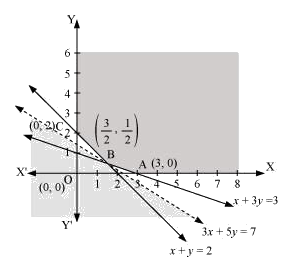Since, the feasible region is unbounded, the corner points of the feasible region are $$A\left( {3,0} \right),B\left( {\frac{3}{2},\frac{1}{2}} \right)$$ and $$C\left( {0,2} \right)$$. The values of $$Z$$ at these corner points are as follows:

 Corner point $$Z = 3x + 5y$$ $${\rm{A}}\left( {3,0} \right)$$ 9 $${\rm{B}}\left( {\frac{3}{2},\frac{1}{2}} \right)$$ 7 $$\to$$Minimum $${\rm{C}}\left( {0,2} \right)$$ 10

As the feasible region is unbounded, therefore, $$7$$ may or may not be the minimum value of $$Z.$$

For this, we draw the graph of the inequality, $$3x + 5y < 7$$, and check whether the resulting half plane has points in common with the feasible region or not.

Since, feasible region has no common point with $$3x + 5y < 7$$

Thus, the minimum value of $$Z$$ is $$7$$ at $$\left( {\frac{3}{2},\frac{1}{2}} \right)$$.

## Chapter 12 Ex.12.1 Question 5

Maximize $$Z = 3x + 2y$$

Subject to $$x + 2y \le 10,\;3x + y \le 15,\;x,\;y \ge 0$$

### Solution

The feasible region determined by the constraints, $$x + 2y \le 10,\;3x + y \le 15$$ and $$x,\;y \ge 0$$, is given by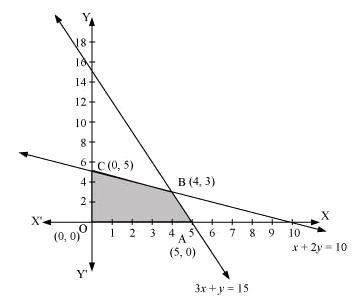Since the corner points of the feasible region are $$A\left( {5,0} \right),\;B\left( {4,3} \right)$$ and $$C\left( {0,5} \right)$$.

The values of $$Z$$ at these corner points are as follows:

 Corner point $$Z = 3x + 2y$$ $${\rm{A}}\left( {5,0} \right)$$ 15 $${\rm{B}}\left( {4,3} \right)$$ 18 $$\to$$ Maximum $${\rm{C}}\left( {0,5} \right)$$ 10

Thus, the maximum value of $$Z$$ is $$18$$ at the point $$B\left( {4,3} \right)$$.

## Chapter 12 Ex.12.1 Question 6

Minimize $$Z = x + 2y$$

Subject to $$2x + y \ge 3,\;x + 2y \ge 6,\;x,\;y \ge 0$$

### Solution

The feasible region determined by the constraints, $$2x + y \ge 3,\;x + 2y \ge 6$$ and $$x,\;y \ge 0$$, is given by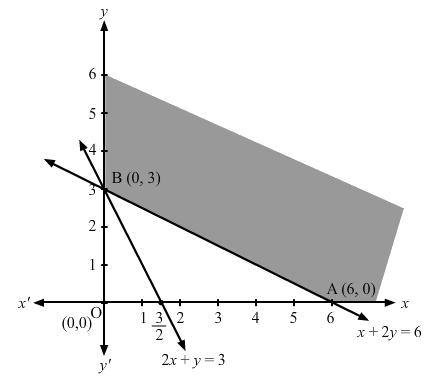Since the corner points of the feasible region are $$A\left( {6,0} \right)$$ and $$B\left( {0,3} \right)$$.

The values of $$Z$$ at these corner points are as follows:

 Corner point $$Z = x + 2y$$ $${\rm{A}}\left( {6,0} \right)$$ 6 $${\rm{B}}\left( {0,3} \right)$$ 6

Since the values of $$Z$$ at points $$A$$ and $$B$$ is same. If we take any other point such as $$\left( {2,2} \right)$$on line $$x + 2y = 6$$, then $$Z = 6$$.

Thus, the minimum value of $$Z$$ occurs for more than 2 points.

Thus, the value of $$Z$$ is minimum at every point on the line, $$x + 2y = 6$$.

## Chapter 12 Ex.12.1 Question 7

Minimize and Maximize $$Z = 5x + 10y$$

subject to $$x + 2y \le 120,\;x + y \ge 60,\;x - 2y \ge 0,\;x,\;y \ge 0$$

### Solution

The feasible region determined by the constraints,$$x + 2y \le 120,\;x + y \ge 60,\;x - 2y \ge 0$$ and $$x,\;y \ge 0$$ is given by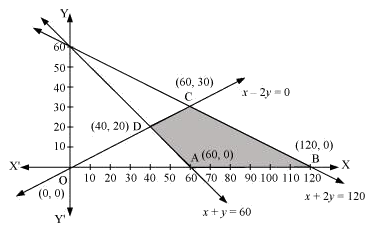Since the corner points of the feasible region are $$A\left( {60,0} \right),{\rm{ }}B\left( {120,0} \right),C\left( {60,30} \right)$$ and $$D\left( {40,20} \right)$$.

The values of $$Z$$ at these corner points are as follows:

 Corner point $$Z = 5x + 10y$$ $${\rm{A}}\left( {60,0} \right)$$ 300 $$\to$$Minimum $${\rm{B}}\left( {120,0} \right)$$ 600 $$\to$$Maximum $${\rm{C}}\left( {60,30} \right)$$ 600 $$\to$$Maximum $${\rm{D}}\left( {40,20} \right)$$ 400

The minimum value of $$Z$$ is $$300$$ at $$A\left( {60,0} \right)$$ and the maximum value of $$Z$$ is $$600$$ at all the points on the line segment joining $$B\left( {120,0} \right)$$ and $$C\left( {60,30} \right)$$.

## Chapter 12 Ex.12.1 Question 8

Minimize and Maximize $$Z = x + 2y$$

Subject to $$x + 2y \ge 100,\;2x - y \le 0,\;2x + y \le 200,\;x,\;y \ge 0$$

### Solution

The feasible region determined by the constraints, $$x + 2y \ge 100,\;2x - y \le 0,\;2x + y \le 200$$ and $$x,\;y \ge 0$$ is given by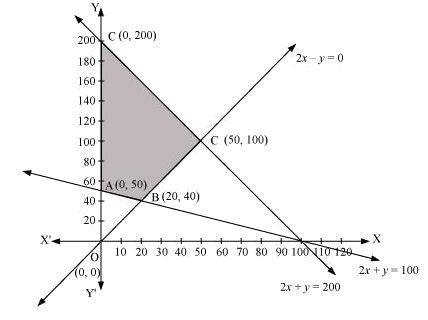The corner points of the feasible region are $${\rm{A}}\left( {0,50} \right){\rm{, B}}\left( {20,40} \right),\;{\rm{C}}\left( {50,100} \right)\;{\rm{and}}\;{\rm{D}}\left( {0,200} \right)$$.

The values of $$Z$$ at these corner points are as follows:

 Corner point $$Z = x + 2y$$ $${\rm{A}}\left( {0,50} \right)$$ 100 $$\to$$Minimum $${\rm{B}}\left( {20,40} \right)$$ 100 $$\to$$Minimum $${\rm{C}}\left( {50,100} \right)$$ 250 $${\rm{D}}\left( {0,200} \right)$$ 400 $$\to$$Maximum

The maximum value of $$Z$$ is $$400$$ at $$D\left( {0,200} \right)$$ and the minimum value of $$Z$$ is $$100$$ at all the points on the line segment joining $$A\left( {0,50} \right)$$ and $$B\left( {20,40} \right)$$.

## Chapter 12 Ex.12.1 Question 9

Maximize $$Z = - x + 2y$$

subject to the constraints: $$x \ge 3,\;x + y \ge 5,\;x + 2y \ge 6,\;y \ge 0$$

### Solution

The feasible region determined by the constraints, $$x \ge 3,\;x + y \ge 5,\;x + 2y \ge 6$$ and $$y \ge 0$$, is given by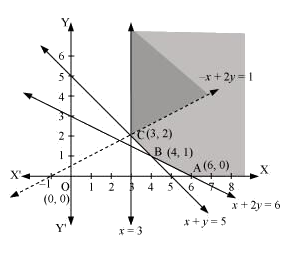Since, the feasible region is unbounded, the values of $$Z$$ at corner points $$A\left( {6,0} \right),B\left( {4,1} \right)$$ and $$C\left( {3,2} \right)$$ are as follows:

 Corner point $$Z = - x + 2y$$ $${\rm{A}}\left( {6,0} \right)$$ $$Z = - 6$$ $${\rm{B}}\left( {4,1} \right)$$ $$Z = - 2$$ $${\rm{C}}\left( {3,2} \right)$$ $$Z = 1$$

As the feasible region is unbounded, therefore, $$Z = 1$$ may or may not be the maximum value.

For this, we graph the inequality, $$- x + 2y > 1$$, and check whether the resulting half plane has points in common with the feasible region or not.

The resulting feasible region has points in common with the feasible region.

Thus, $$Z = 1$$ is not the maximum value.

Therefore, $$Z$$ has no maximum value.

## Chapter 12 Ex.12.1 Question 10

Maximize $$Z = x + y$$

Subject to $$x - y \le - 1,\; - x + y \le 0,\;x,\;y \ge 0$$

### Solution

The feasible region determined by the constraints,$$x - y \le - 1,\; - x + y \le 0$$ and $$x,\;y \ge 0$$, is given by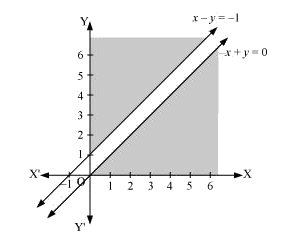There is no feasible region and thus, $$Z$$ has no maximum value.

Instant doubt clearing with Cuemath Advanced Math Program